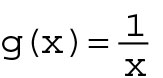##### Pre-Calculus For DummiesFunctions can be categorized in many different ways. Here, you see functions in terms of the operations being performed. Here, though, you see classifications that work for all the many types of functions. If you know that a function is even or odd or one-to-one, then you know how the function can be applied and whether it can be used as a model in a particular situation. You’ll have a heads-up as to how the function behaves in different circumstances.

## Even and odd functions

Knowing whether a function is even or odd helps you to graph it because that information tells you which half of the points you have to graph. These types of functions are symmetrical, so whatever appears in one half is exactly the same as the other half. If a function is even, the graph is symmetrical over the y-axis. If the function is odd, the graph is symmetrical about the origin.
• Even function: The mathematical definition of an even function is f(–x) = f(x) for any value of The simplest example of this is f(x) = x2; f(3) = 9, and f(–3) = 9. Basically, the opposite input yields the same output. Visually speaking, the graph is a mirror image across the y-axis.
• Odd function: The definition of an odd function is f(–x) = –f(x) for any value of The opposite input gives the opposite output. These graphs have 180-degree symmetry about the origin. If you turn the graph upside down, it looks the same. For example, f(x) = x3 is an odd function because f(3) = 27 and f(–3) = 27.

## One-to-one functions

A function is considered to be one-to-one if every output value is unique — it appears just once in the range. Another way of saying this is that every input value has exactly one output value (which is essentially the definition of a function), and every output value comes from exactly one input value. There are no repeats in output values. Examples of one-to-one functions are f(x) = 2x3 andOne-to-one functions are the only type of functions that have inverses.